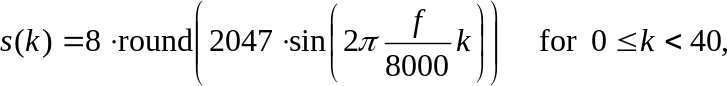## 8.2.6 CTM modulator

26.2263GPPCellular text telephone modemGeneral descriptionRelease 17TS

The bit-stream coming from the interleaver is grouped in pairs of two bits. Each pair of two bits is modulated into a sine waveform of length 5 ms (40 samples) starting with a phase value of zero. The relation between the bits and the modulated waveform is as follows:where it is assumed that the audio samples are PCM‑coded with 2’s complement representation and with a resolution of 13 significant bits, left‑justified in a 16‑bit word. The three least significant bits are set to ‘0’. The amplitude is set to a value lower than maximum in order to avoid saturation of speech codecs within the transmission path.

Depending on the values of the bits bit0/bit1, one of the frequencies 400 Hz, 600 Hz, 800 Hz, and 1000 Hz is used, provided that at least one of the two bits is not marked as to be muted. In case that both bits bit0 and bit1 are marked to be muted, a sequence of 40 zero‑valued samples is generated.

The output signal is also zero in case that no valid bits are available at the CTM modulator’s input.

Table 1: Frequency parameter f for the CTM modulator

Hz

 bit1=0 bit1=1 bit1=mute bit0=0 400 600 600 bit0=1 800 1000 1000 bit0=mute 800 1000 0